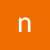This is a user generated content for MyStory, a YourStory initiative to enable its community to contribute and have their voices heard. The views and writings here reflect that of the author and not of YourStory.# How Do You Compare Pure Python, NumPy, and TensorFlow?Tuesday January 15, 2019,

Today, technology invention has to make things happen in an unexpected way. In the olden days, learning new things was a challenging task, and people feared to change from one technology to another. Today, people are ready to adopt new technology in a short span of time, and a lot of young people would like to learn to trend programming languages like python. But how do you compare pure python, NumPy, and Tensor Flow?

We do have many programming languages to solve a problem. And all these ways gives you the result. But the amount of time, it needs to run the problem matters. As a fresher to the python libraries like Tensor Flow, you people do not find any differences between the time taken for running in various languages like NumPy, pure python etc. But when you start doing the large project the actual difference among those can be seen.

How Do You Compare Pure-Python and NumPy?

Before going to the comparison of these libraries, let me first define what they actually mean

Pure Python

If your python package does not contain any python code, it is called pure python, and a standalone package is necessary.

NumPy

It provides support for large matrices and multi-dimensional arrays. In addition, it contains a collection of mathematical functions to operate these elements. The project relies on well-known packages implemented in other languages to perform efficient computations. NumPy brings both the python expressiveness and MATLAB similar performance.

If you are interested to learn python please go through python online training

Tensor Flow

For numerical computation, it is an open-source library. Google brain team researchers and engineers developed this library functions. The main focus of the library is to provide easy to use API to implement practical algorithms. And to deploy them using CPU’s, GPU (or) a cluster.

Test Data

Let's compare the three library functions using the test data. To test the library performance, you need to consider the two-parameter linear regression problem. This model has the intercept term, w_0 and the single coefficient w_1.

Initially, consider the N pairs of Inputs ‘x’ and the desired outputs.’ Its idea is to model the relationship between the inputs and outputs using a linear model y = w _0 + w _1 *x (here, the output y is approximately equal to the desired output for every pair ( x.d).

Consider the above code for the purpose of the test data.

This program creates a set of 10000 inputs * linearly distributed over the interval from 0 to 2. It can create the desired inputs d = 3 + 2 *x + noise. Here Gaussian normal distribution applied for noise and zero mean and the standard deviation sigma = 0.1

So by creating the x and d in this way, you are effectively stipulating the optimal solution for the w_ 0 and w _ 1 as 3 and 2 respectively. And to fit this training data set python online training to suggest several methods to estimate the w_ 0 and W_ 1. And ordinary least squares is the well-known method for the estimation of W _ 0 and W _ 1. Here we can minimize the square of the error.

Let us find out the values in the pure python.

The python function above estimate the parameters of w_0 and w_1 using the gradient descent. And before running through each epoch empty containers of zeros are initialized for y, w, and grad. At each epoch after the update output of the model is calculated. Using list comprehensions vector operations are perfumed. Using the time library the algorithm elapsed time is measured. It takes 18.65 seconds to estimate the values of w_ 0 and w_ 1.

Using NumPy

At a blazing speed operations are performed. This is done by the projects like BLAS, LAPACK for underlying the implementation. This takes the advantage of vectored operations with NumPy arrays. Here, you can notice that there are alternate ways to solve the given problem. For example, you can simplify the f*err. Here x is a 2 D array. And this includes a column vector of 1 ’s. time it. Repeats return a list. Actually, this is not an efficient problem, because, it requires the dot product of entre ones with another vector. Time. The list gives a time takes for each iteration of the loop. And the average gives you the time complexity of the loop.

Tensor Flow

For numerical computations, it is an open-source library. Python API Tensor Flow implements the graph computations.

Nodes in the graph represent the mathematical operations and the graph represents the multidimensional arrays communicated between them.

A Tensor Flow takes the graph of computations, using optimized C ++ code. Using this graph, we can able to identify the operations that can be run in parallel.

For timing operations, it uses the same timing algorithm a NumPy. This would roughly take 1.2 seconds to calculate both w_ 0 and w_ 1.

So by this, the developer/designer needs to think regarding the selection of the algorithm.

So like this, we can select the algorithm as per our requirement and do as follows. And many developers consider the problem solved using this feature. So have knowledge over all the algorithms to select the best.

Share on